Name:    Geometry Chapter 4 Practice MC

1.

Classify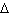LMN.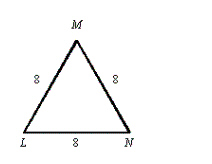a. Isosceles b. Equilateral c. none of these d. Scalene

2.

Name a right triangle.a. none of these b.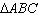c.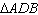d.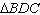3.

Find the value of x.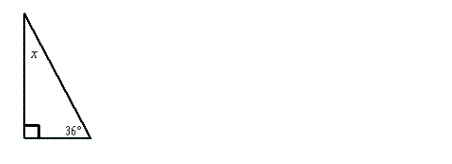a.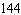° b.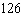° c.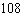° d.°

4.

The two triangle-shaped gardens are congruent. Find the missing side lengths and angle measures.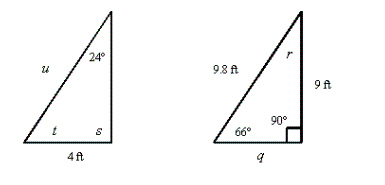a. q = 4 ft; r = 24°; s = 90°; t = 66°; u = 9.8 ft b. q = 9 ft; r = 24°; s = 90°; t = 66°; u = 9.8 ft c. q = 4 ft; r = 24°; s = 66°; t = 90°; u = 4 ft d. q = 4 ft; r = 24°; s = 90°; t = 66°; u = 4 ft

5.

Refer to the figure shown. Which of the following statements is true?a.c.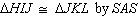b.d.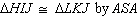6.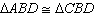. Name the theorem or postulate that justifies the congruence.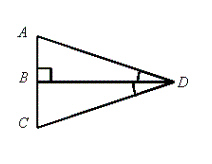a. SAS b. AAS c. ASA d. HL

7.. Name the theorem or postulate that justifies the congruence.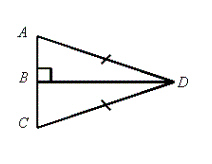a. SAS b. ASA c. AAS d. HL

8.. Name the theorem or postulate that justifies the congruence.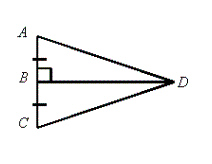a. SAS b. HL c. ASA d. AAS

9.

Find the value of x:a. 35° b. 86° c. 129° d. 145°

10.

Find the value of x: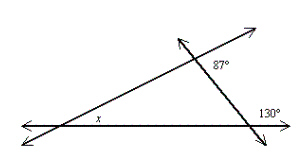a. 143° b. 93° c. 37° d. 130°

11.

What is the measure of each base angle of an isosceles triangle if its vertex angle measures 36 degrees and its 2 congruent sides measure 14 units?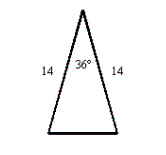a. 54° b. 144° c. 36° d. 72°

12.

Find the values of x and y.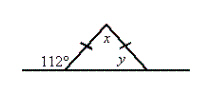a. x = 68°, y = 112° c. x = 44°, y = 112° b. x = 44°, y = 68° d. x = 68°, y = 48°